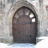QlikView App Dev

Discussion Board for collaboration related to QlikView App Development.

Announcements
QlikWorld 2022, LIVE in Denver CO., May 16-19, 2022. REGISTER NOW TO RECEIVE EARLY BIRD PRICING
cancel
Showing results for
Did you mean:Creator III

Load variables from file that contain variables

Hello there,

I already use the method outlined here by Barry Harmsen:

http://www.qlikfix.com/2011/09/21/storing-variables-outside-of-qlikview/

However, I have run into an issue where I have a need for a variable to be loaded based on the value of another variable. Let me explain what I mean.

Lets assume I have these two variables:

vMedClaim = 1;

vDrillNo = IF(\$(vMedClaim) = 1, '<250',250);

What I want to occur, during the script refresh, is for vDrillNo to either be '<250' or 250 as determined by the vMedClaim variable. However when I follow the method outlined in Barry's blog, the full expression is stored as the value of vDrillNo even though I am using the LET command.

Thoughts?

1 Solution

Accepted SolutionsMVP

I have not heard of that, but I barely use variables, so might have heard of it and then forgot about it.... anyways try now

variable

 IF(\$(vMedClaims) = 1,'<250','250')

Expression

=Sum({<Event_ServiceCat = {"\$(=\$(vDrilldownSvcCat))"}>}Dollars)

28 RepliesMVP

Both vMedClaim and vDrillNo are part of the Excel file variables?Creator III
Author

No.

vMedClaim comes from an include file that is loaded previously.Specialist II

Hi,

example :

let v1 = 1;

let v2 = 'if(\$(v1)<5,''Z'',''W'')';  //  here use twice simple quote

let v = \$(v2);so for you :

vDrillNo = \$(IF(\$(vMedClaim) = 1, ''<250'',''250'')

\$ if you want to interprete value in script,and two simple quote, if not blank

regardsCreator III
Author

Unfortunately this doesn't work for me.

I used single quotes around the values, (2 on each side as described).

Here is what my variable looks like once loaded into the application (the same as in excel).Creator III
Author

Assume vMedClaim = 1

The desired stored value of vDrillNo = '<250'

Ultimately I am trying to use vDrillNo inside set analysis and it doesnt evaluate the way I would expect.MVP

Would you be able to create a mock up of what you have?Specialist II

I thought you evaluate variable in script,

if it is in front :

in script :

let v1 = 1;

let v2 = 'if(\$(v1)<5,''Z'',''W'')';don't put \$ in definition of variable, but evaluate in front endCreator III
Author

Yes that might be easiest.

Note - In these files the variables are vMedClaims and vDrilldownSvcCat (instead of vDrillNo).

My goal is for vDrilldownSvcCat to be loaded into qlikview having the expression evaluated (so it returns either the <250 or 250) as I am trying to use \$(vDrilldownSvcCat) in the following set analysis:

=Sum({<Event_ServiceCat =\$(vDrilldownSvcCat)>}Dollars)

TIAMVP

See if this works for you

=Sum({<Event_ServiceCat = {"\$(vDrilldownSvcCat)"}>}Dollars)Community Browser